# Prerequisites

RaceID is a method for cell type identification from single-cell RNA-seq data by unsupervised learning. An initial clustering is followed by an outlier identification based on a backgorund model of combined technical and biological variability in single-cell RNA-seq data obtained by quantification with unique molecular identifiers. StemID permits subsequent inference of a lineage tree based on clusters, i.e. cell types, identified by RaceID. The current version of RaceID (RaceID3) and StemID (StemID2) are published (Herman, Sagar, and Grun 2018). This package implements additional improvements in rare cell type detection, offers batch effect removal utilities, optional imputing of gene expression, and substantially decreases runtime as well as memory usage. We tested the method successfully on a dataset of ~50k cells. We now added another method termed VarID (Grun 2020) to the package, which allows the quantification of local gene expression variability, but furthermore offers an alternative clustering approach to RaceID based on density clustering which has certain advantages and, in particular, permits handling much larger datasets, since there is no requirement of storing a distance matrix. This method can be fully integrated into a RaceID analysis (see section "VarID"). RaceID offers several optional steps and here we will show examples of how to perform typical RaceID/StemID analyses.

# RaceID

Input to RaceID is a matrix of raw expression values (unique molecular identifiers with or without Poisson correction (Grun, Kester, and van Oudenaarden 2014)) with cells as column and genes as rows. This matrix can be provided as a matrix object, a data.frame or a sparse matrix produced by the `Matrix` package.

The `RaceID` package comes with sample data `intestinalData` for murine intestinal epethelial cells stored in sparse matrix format. The dataset was published previously (Grun et al. 2016).

RaceID and StemID functions have various input and output parameters, which are explained in detail on the `help` pages. Here, we mostly use default parameters, which represent a good choice for common datasets.

To start the analysis, a RaceID single-cell sequencing (`SCseq`) object is initialized with a count matrix.

``````library(RaceID)
sc <- SCseq(intestinalData)``````

The first step is the application of filtering for the purpose of quality control. Cells with a relatively low total number of transcripts are discarded.

``sc <- filterdata(sc,mintotal=2000)``

In this example, we filter out cells with <2,000 transcipts. The function allows further filtering of genes by choosing the input parameters `minexpr` and `minnumber`, i.e. only genes with at least `minexpr` transcripts in at least `minnumber` cells are retained. The filtered and normalized expression matrix (normalized to the minimum total transcript count across all cells retained after filtering) can be retrieved by the `getfdata` function:

``fdata <- getfdata(sc)``

The `filterdata` function allows the detection and removal of batch effects by different methods as outlined below in addtional examples. Alternatively, individual genes or groups of genes correlating to specific genes can be removed with the `FGenes` and `CGenes` arguments. This frequently allows a minimally invasive removal of batch effects or of particularly highly expressed genes with an unwanted dominating effect on the clustering.

Next, the distance matrix is computed as the input for clustering and outlier identification. This can be done with or without imputing gene expression from nearest neighbours (see example below for imputing). RaceID offers various alternative metric, e.g. spearman, kendall, and euclidean, as well as measure of proportionality (rho and phi from the `propr` package).

``sc <- compdist(sc,metric="pearson")``

This function computes the distance matrix based on highly variable genes by default. If all genes should be used, then the parameter `FSelect` needs to be set to `FALSE`. On this distance matrix clustering is performed:

``sc <- clustexp(sc)``

To infer the initial cluster number, this function computes the average within-cluster dispersion up to a number of clusters specified by the `clustnr` arguments, which equals 30 by default. If more major populations are expected, this parameter needs to be set to higher values which will increase the run time. The initial cluster number only serves as a rough estimate, since the subsequent outlier identification will infer additional clusters. The internal inference of the cluster number and the evaluation of cluster stability by the computation of Jaccard's similarity is done on all cells by default. For large datasets it is reasonable to subsample a limited number of cells, by setting the `samp` argument, e.g., to 1,000. In this case, the cluster number is inferred based on this smaller subset and Jacccard's similarity is not computed by bootstrapping but by sub-sampling. For k-medoids clustering, subsetting will imply almost deterministic clustering partitions, if the sample size approaches the size of the dataset. Therefore, `samp` should be signicantly smaller then the size of the dataset. Otherwise, bootstrapping is better for assessing the cluster stability. Subsampling can be expected to give a good estimate of the number of major clusters. Additional small clusters which might have been missed by the sampling can be reintroduces during the outlier identification step.

The inferred cluster number can be inspected in a saturation plot, which shows the decrease of the average within-cluster dispersion with increasing cluster number. If this decrease becomes constant, saturation is reached. The automatically chosen cluster number is detected such that the decrease is equal to the decrease upon further increasing the cluster number within the error bars:

``plotsaturation(sc,disp=FALSE)``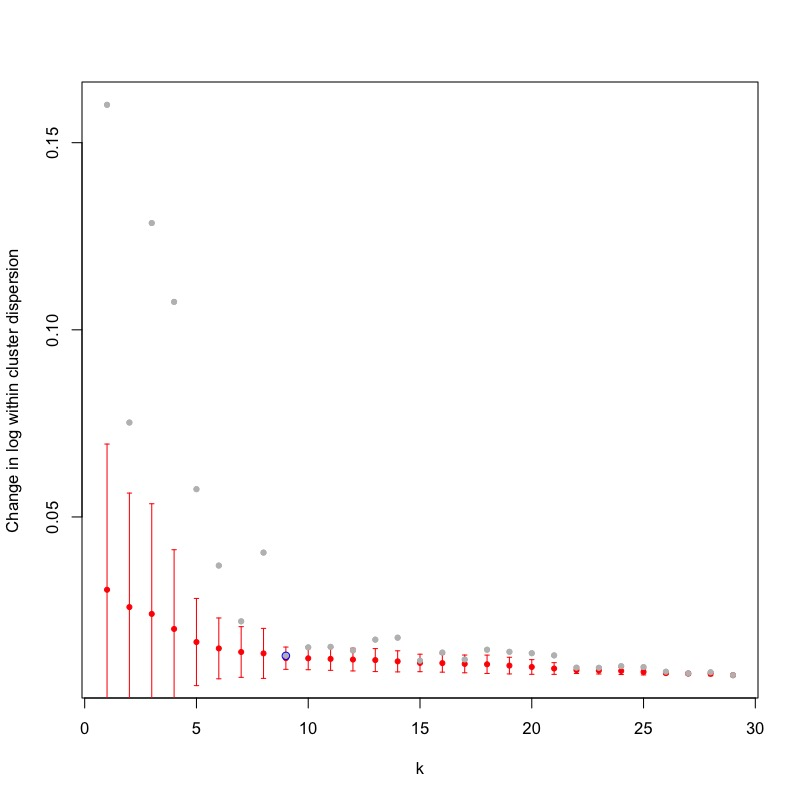The average within-cluster dispersion can also by plotted:

``plotsaturation(sc,disp=TRUE)``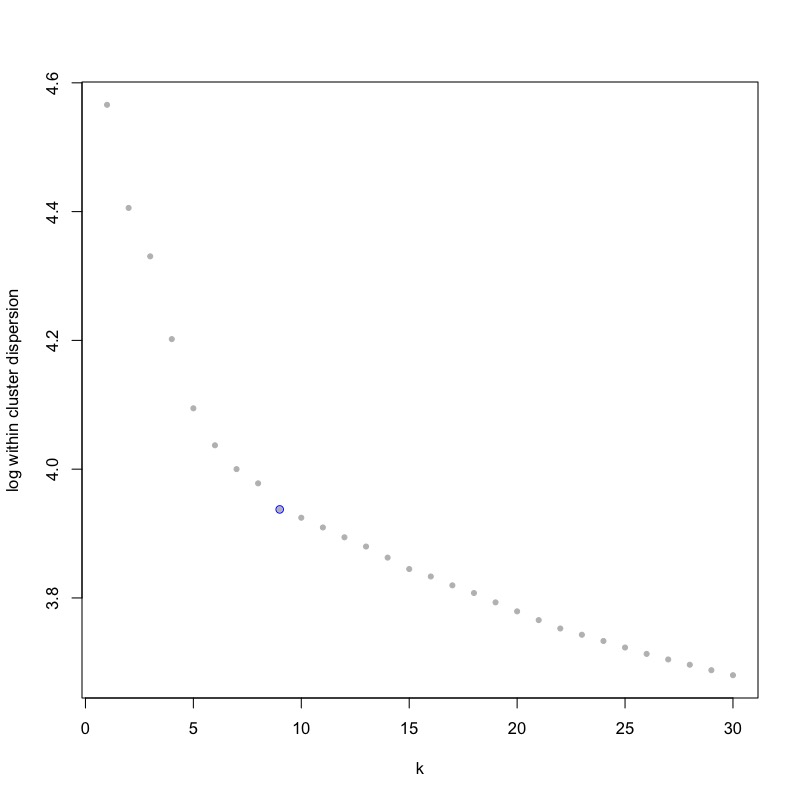The cluster stability as assessed by Jaccard's similarity should also be inspected:

``plotjaccard(sc)``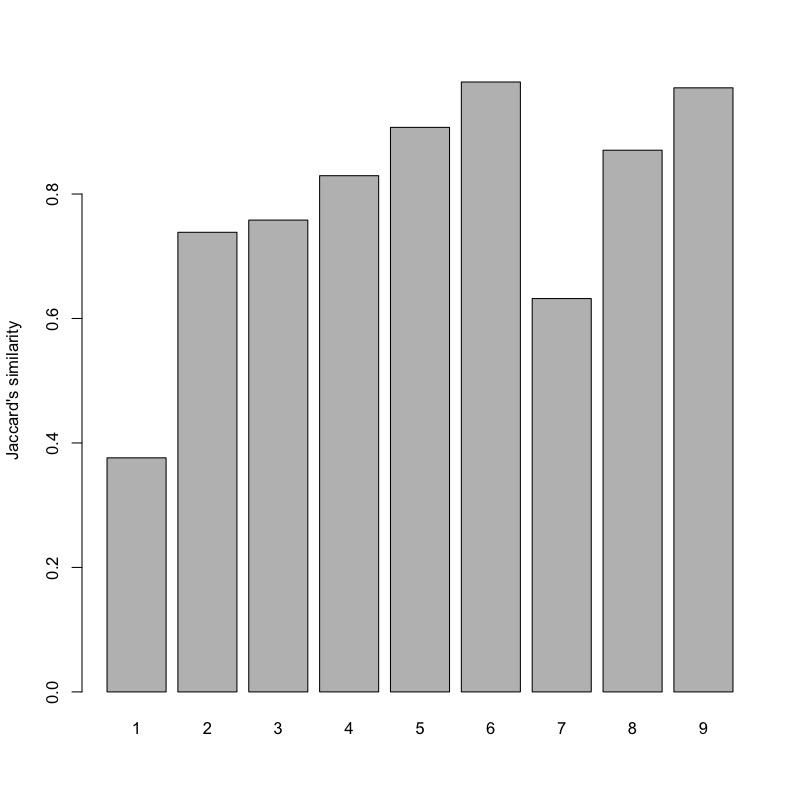In this example, the automated criterion overestimated the cluster number leading to instability as indicated by low Jaccard's similarity. Based on visual inspection of the average within-cluster dispersion as a function of the cluster number, we manually set the cluster number to 7 without recomputing the saturation behaviour.

``sc <- clustexp(sc,cln=7,sat=FALSE)``

This function perform k-medoids clustering by default. K-means clustering or hierarchical clustering can be chosen with the `FUNcluster` argument. For very large datasets, hierarchical clustering leads to significantly smaller run time.

Subsequently, outliers in the initial k-medoids clusters are identified based on an internally computed background model for the expected gene expression variability and the assumption that transcript counts follow a negative binomial distribution defined by the mean and the variance of the expression of each gene in a cluster. Outlier genes will be in the tail of this distribution at a p-value defined by the `probthr` parameter (1e-3 by default), and outlier cells require the presence of a number of outlier genes defined by the `outlg` parameter (2 by default).

``sc <- findoutliers(sc)``

In contrast to previous versions, outlier genes are inferred from non-normalized transcript counts, which follow a negative binomial distribution modeling the joint technical and biological variability. The assumption of a negative binomial distribution was demonstrated for raw transcript (UMI) count data, but is not strictly valid for normalized expression values (Grun, Kester, and van Oudenaarden 2014). Hence, RaceID does not require normalization, since the correlation-based metric for the computation of the distance object is also independent of the normalization. Normalizaion is only relevant when using, e.g., the euclidean metric for the derivation of the distance matrix. RaceID applies a simple size normalization for data representation and follow-up analyses.

The background noise model can be inspected:

``plotbackground(sc)``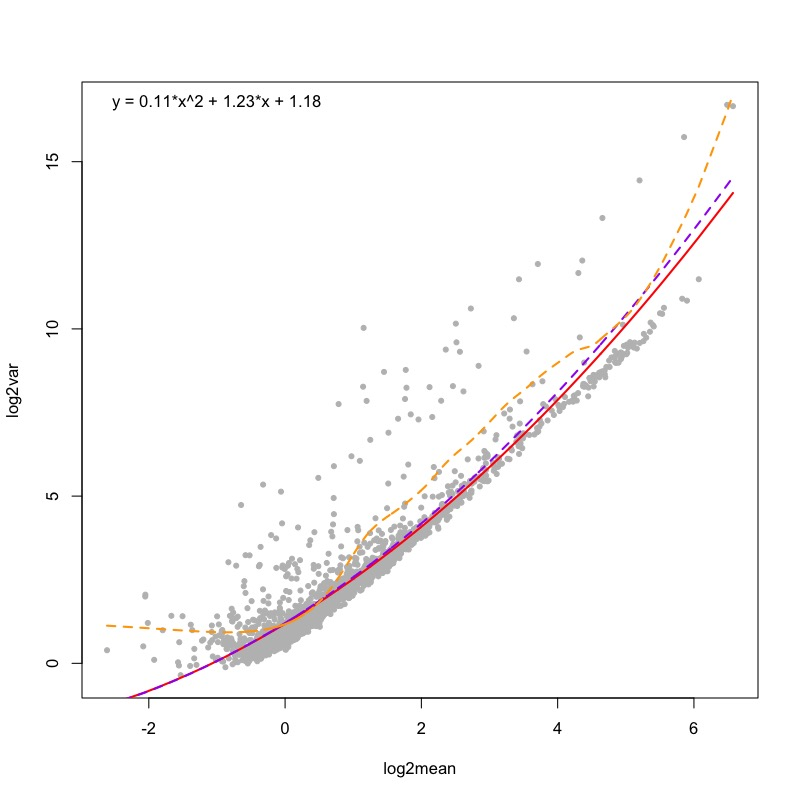The number of outliers as a function of the p-value can be plotted:

``plotsensitivity(sc)``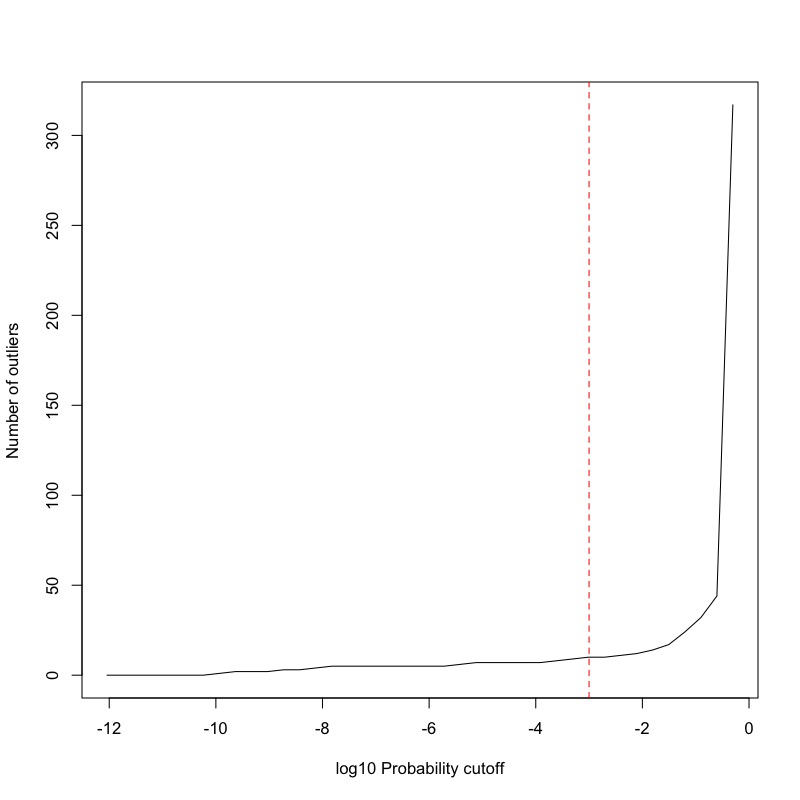Another way of checking the presence of outliers is the inspection of a barplot of p-values across all cells in each cluster:

``plotoutlierprobs(sc)``